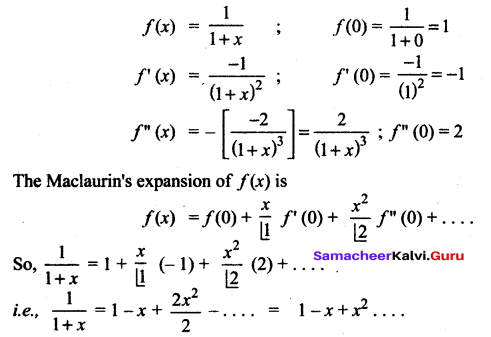# Samacheer Kalvi 12th Maths Solutions Chapter 7 Applications of Differential Calculus Ex 7.4

## Tamilnadu Samacheer Kalvi 12th Maths Solutions Chapter 7 Applications of Differential Calculus Ex 7.4

Question 1.
Write the Maclaurin series expansion of the following functions:
(i) ex
(ii) sin x
(iii) cos x
(iv) log (1 – x); -1 ≤ x < 1
(v) tan-1 (x) ; -1 ≤ x ≤ 1
(vi) cos2 x
Solution: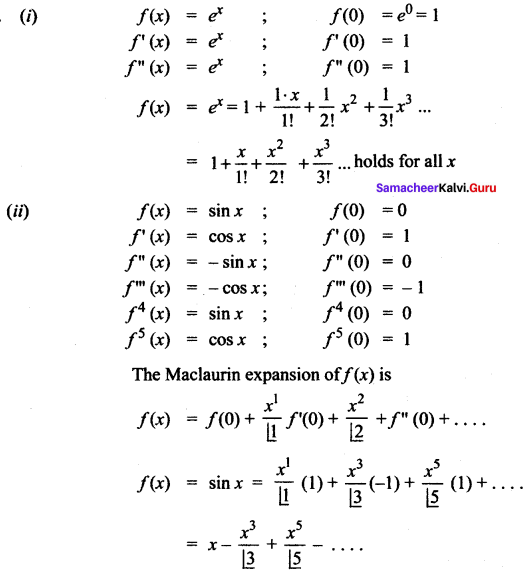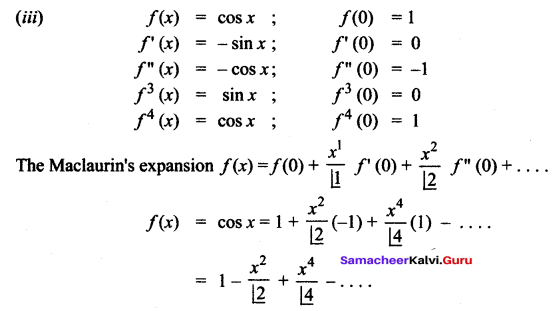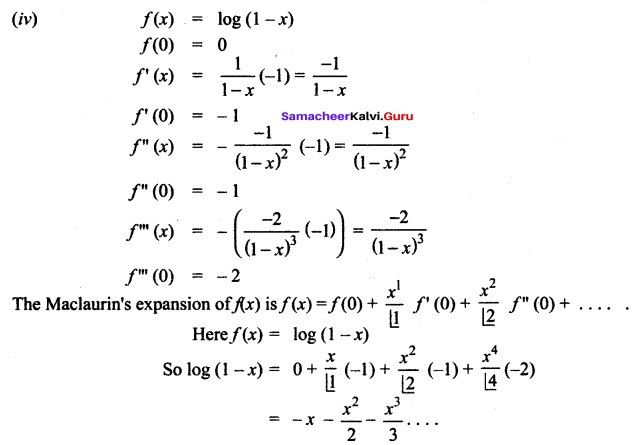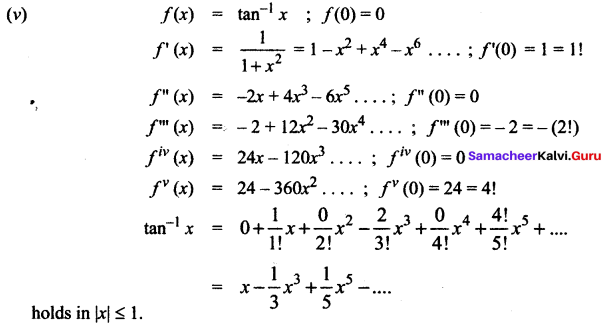(vi) f(x) = cos2 x
f(0) = 1
f'(x) = 2 cos x (- sin x) = – sin 2x
f'(0) = 0
f”(x) = (-cos 2x)(2)
f”(0) = -2
f”'(x) = -2[- sin 2x](2) = 4 sin 2x
f”'(0) = 0
f4 (x) = 4(cos 2x)(2) = 8 cos 2x
f4 (0) = 8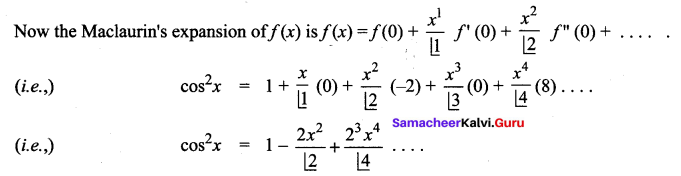Question 2.
Write down the Taylor series expansion, of the function log x about x = 1 upto three non-zero terms for x > 0.
Solution: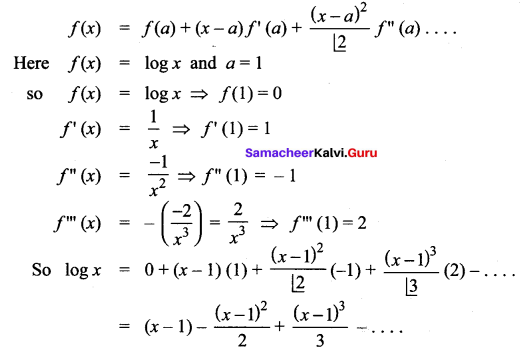Question 3.
Expand sin x in ascending powers x – $$\frac{\pi}{4}$$ upto three non-zero terms.
Solution:
f (x) = sin x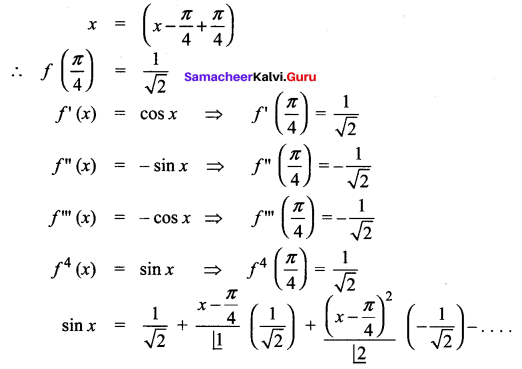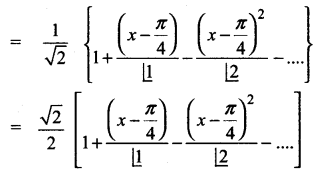Question 4.
Expand the polynomial f(x) = x2 – 3x + 2 in powers of x – 1
Solution:
f(x) = x2 – 3x + 2 = (x – 1) (x – 2)
f(1) = 0
f'(x) = 2x – 3 ; f'(1) = -1
f”(x) = 2 ; f”(1) = 2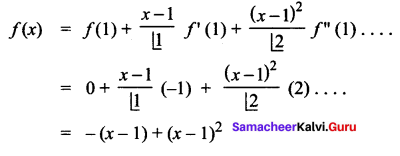### Samacheer Kalvi 12th Maths Solutions Chapter 7 Applications of Differential Calculus Ex 7.4 Additional Problems

Question 1.
The Taylor’s series expansion of f(x) = sin x about x = $$\frac{\pi}{2}$$ is obtained by the following way.
Solution: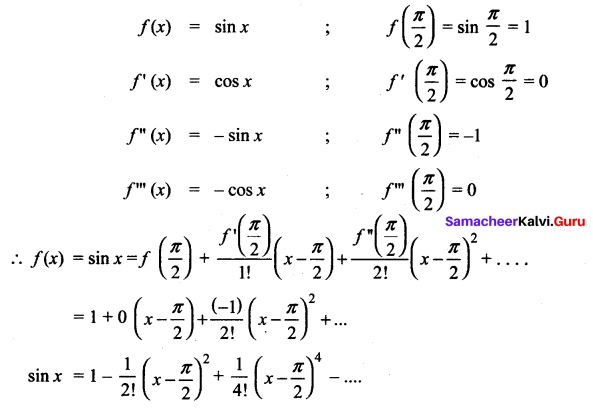Question 2.
Obtain the Maclaurin’s series expansion for the following functions.
(i) ex
(ii) sin2 x
(iii) $$\frac{1}{1+x}$$
Solution:
(i)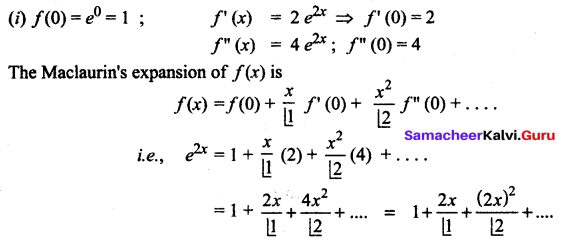(ii)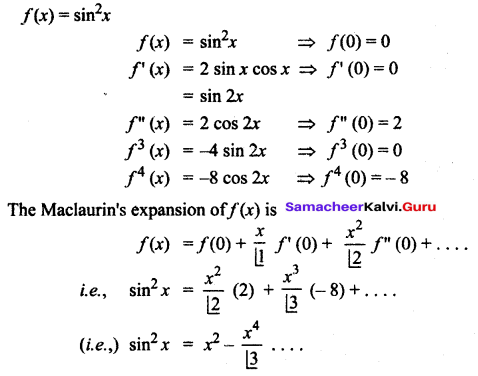(iii)